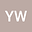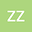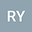loading page

Ground state sign-changing solution for Schr\”{o}dinger-Poisson system with critical growth
•••• Ying Wang ,
• Ziheng Zhang,
• Rong Yuan
Ying Wang
Beijing Normal University
Author ProfileZiheng Zhang
Tiangong University
Author ProfileRong Yuan
Beijing Normal University
Author Profile## Abstract

This article is devoted to study the nonlinear Schr\”{o}dinger-Poisson system with pure power nonlinearities $$\left\{\begin{array}{ll} -\Delta u+u+ \phi u=|u|^{p-1}u+|u|^4u, &x\in \mathbb{R}^3, \\[0.3cm] -\Delta\phi= u^2, &x\in \mathbb{R}^3, \end{array} \right.$$ where $4< p<5$. By employing constraint variational method and a variant of the classical deformation lemma, we show the existence of one ground state sign-changing solution with precisely two nodal domains, which complements the recent work of Wang et al. \cite{Wang2019}.